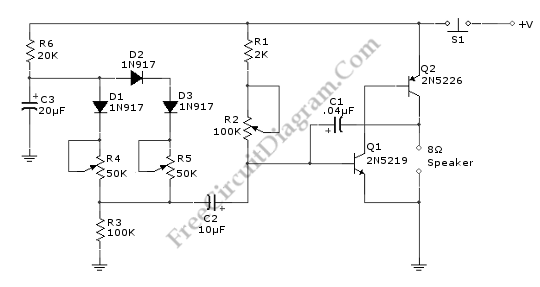## Discrete Sliding Tone (Frequency Ramp) DoorbellThis doorbell circuit produces a low tone that will slide up to higher frequency. The equivalent total resistance connected between the base of Q1 and ground (Rbg) , and coupling capacitor  C1  determines the AF oscillator’s frequency. The resistance (Rbg) is equal to (R2+R1)R3.  Here is the schematic diagram of the circuit: The R2 is used to set the initial […]